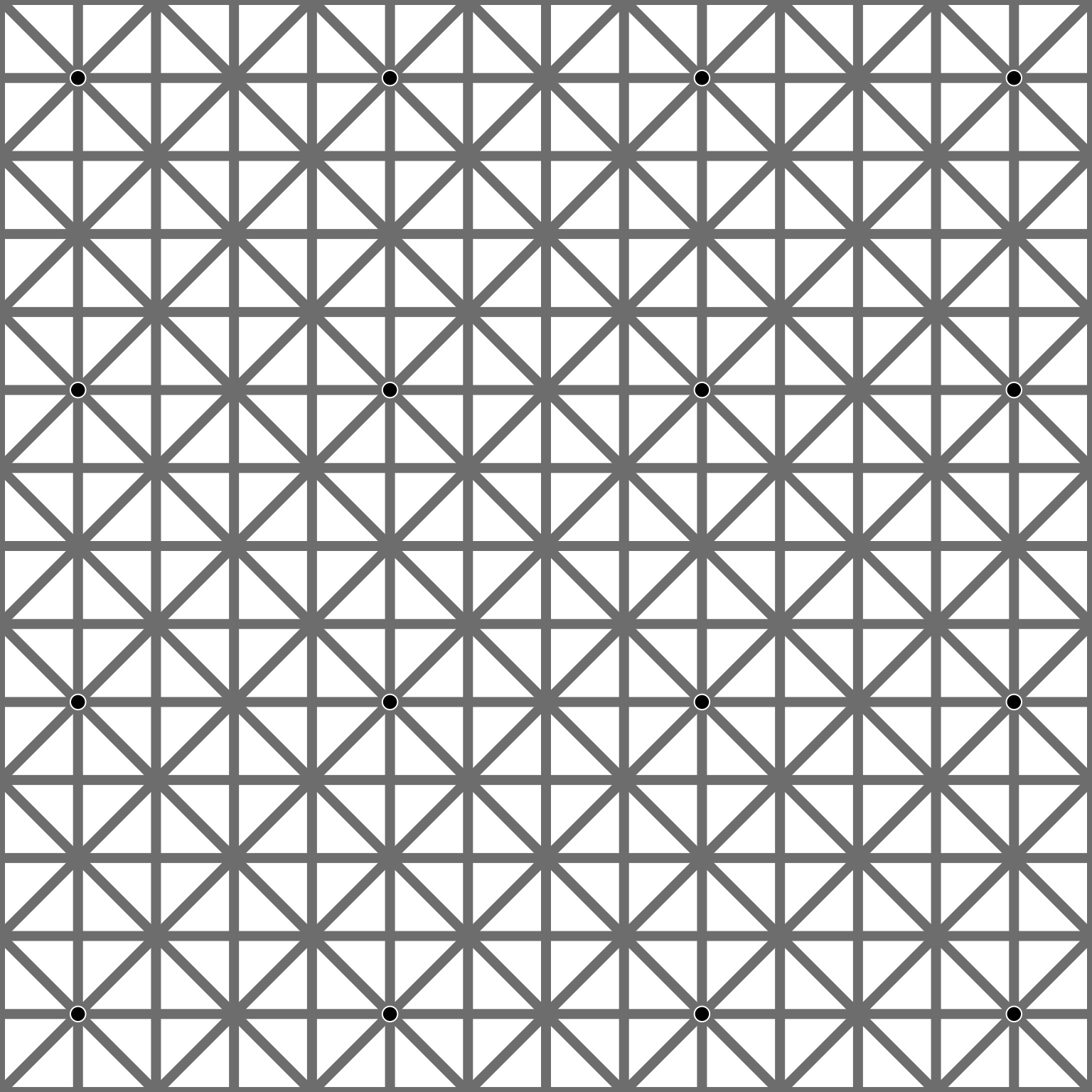Originally created by Dr. Ninio:

You can find some additional information at Sekai No Kitaoka’s website:

library(grid)
# Define
N = 15
c0z = seq(0, 1, length.out = N)
c0 = data.frame(expand.grid(m = 0:1, n = c0z),
i = rep(seq_len(N), each=2))
c1z = seq(-1, 1, length.out = N)
c1 = data.frame(x = c(c1z, c1z+1),
y1 = rep(0:1, each = N),
y2 = rep(1:0, each = N),
i = rep(seq_len(N), 2))
p0z = c0z[seq(2, N, by=4)]
p0 = expand.grid(x=p0z, y=p0z)
# Draw
gpl = gpar(lwd = 7, col = rgb(0.5, 0.5, 0.5))
grid.polyline(c0$m, c0$n ,c0$i, gp = gpl) grid.polyline(c0$n, c0$m ,c0$i, gp = gpl)
grid.polyline(c1$x, c1$y1, id = c1$i, gp = gpl) grid.polyline(c1$x, c1$y2, id = c1$i, gp = gpl)
grid.circle(p0$x, p0$y, r = 0.007, gp = gpar(fill = "black", col = "white", lwd = 1))